# Basic Arithmetic : Creating Equations with Whole Numbers

## Example Questions

### Example Question #1 : Linear Equations With Whole Numbers

Jimmy had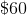in lunch money for school. Everyday he spends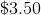for food and drinks. What is the expression that shows how much money will he have after each day, whereis the days, and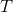is the total amount of money left?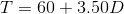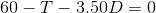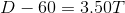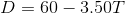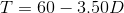Explanation:

Jimmy starts off with $60, and spends$3.50 everyday.

This means that he will have $56.50 after day 1,$53 after day 2, and so forth.

Only one equation satisfies this scenario.  The rest are irrelevant.### Example Question #261 : Equations / Inequalities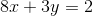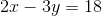What is the solution offor the systems of equations?Explanation:

We add the two systems of equations:

For the Left Hand Side: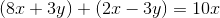For the Right Hand Side: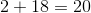So our resulting equation is: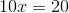Divide both sides by 10:

For the Left Hand Side: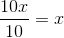For the Right Hand Side: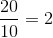Our result is:### Example Question #1 : Linear Equations With Whole Numbers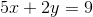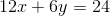What is the solution ofthat satisfies both equations?Explanation:

Reduce the second system by dividing by 3.

Second Equation:We this by 3.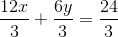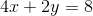Then we subtract the first equation from our new equation.

First Equation:First Equation - Second Equation:

Left Hand Side: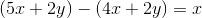Right Hand Side: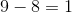Our result is:### Example Question #2 : Linear Equations With Whole Numbers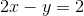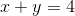What is the solution offor the two systems of equations?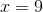Explanation:

We first add both systems of equations.

Left Hand Side: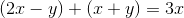Right Hand Side: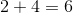Our resulting equation is: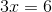We divide both sides by 3.

Left Hand Side: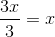Right Hand Side: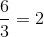Our resulting equation is:### Example Question #51 : Systems Of Equations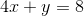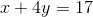What is the solution offor the two systems?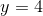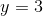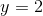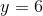Explanation:

We first multiply the second equation by 4.

So our resulting equation is: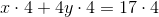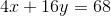Then we subtract the first equation from the second new equation.

Left Hand Side: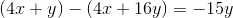Right Hand Side: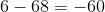Resulting Equation: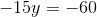We divide both sides by -15

Left Hand Side: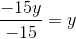Right Hand Side: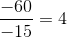Our result is:### Example Question #1 : Linear Equations With Whole Numbers

Dr. Jones charges a $50 flat fee for every patient. He also charges his patients$40 for every 10 minutes that he spends with him. If Mrs. Smith had an appointment that lasted 30 minutes, how much did she have to pay Dr. Jones?

$170$120

$200$90

\$170

Explanation:

We can express Dr. Jones's rate in a linear equation: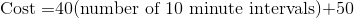Since Mrs. Smith's appointment lasted 30 minutes, we have 3 10-minute intervals. Then, we can plug in that number into our above equation to find out how much the appointment cost.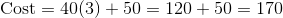### Example Question #1 : How To Find The Whole From The Part

Roman is ordering uniforms for the tennis team. He knows how many people are on the team and how many uniforms come in each box. Which equation can be used to solve for how many boxes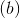Roman should order?

b = number of boxes ÷ number of uniforms per box

b = number of students x number of uniforms per box

b = number of students - number of uniforms per box

b = number of students ÷ number of uniforms per box

b = number of boxes x number of uniforms per box